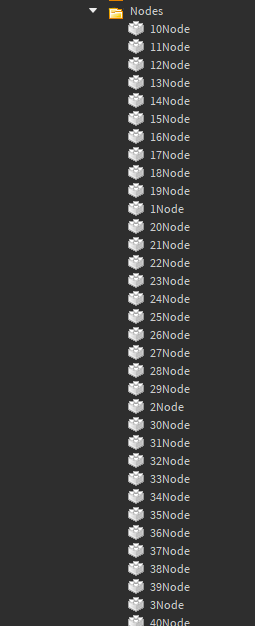# If Condition not working

Hello, I got this folder filled with these nodes:and I’m trying to get a table of these nodes in order using the numbers in their names
so I made this local script:

``````Nodes = Target.Nodes:GetChildren()

local InOrderNodes = {}

for P = 1,#Nodes,1 do
for i = 1,#Nodes,1 do
local str = Nodes[i].Name
local Num = string.gsub(str, "%D+", "")
print(Num,P)

if Num == P then
table.insert(InOrderNodes,Nodes[i])
end

end
end
``````

after having done some testing I noticed that everything works smoothly until it gets to the “if condition”
it doesn’t work even when Num is equal to P!
and I can’t see where is the problem here…

1 Like

Try

``````if tonumber(Num) == P then

end``````
3 Likes

Thank You! this thing finally worked!

1 Like